# Triakis icosahedron

Triakis icosahedron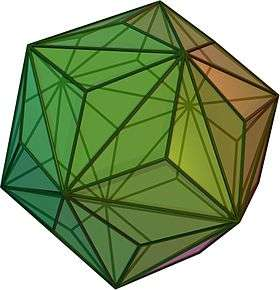TypeCatalan solid
Coxeter diagramConway notationkI
Face typeV3.10.10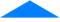isosceles triangle
Faces60
Edges90
Vertices32
Vertices by type20{3}+12{10}
Symmetry groupIh, H3, [5,3], (*532)
Rotation groupI, [5,3]+, (532)
Dihedral angle160°36′45″
arccos(−24 + 155/61)
Propertiesconvex, face-transitive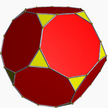Truncated dodecahedron
(dual polyhedron)Net

In geometry, the triakis icosahedron (or kisicosahedron) is an Archimedean dual solid, or a Catalan solid. Its dual is the truncated dodecahedron.

## Orthogonal projections

The triakis icosahedron has three symmetry positions, two on vertices, and one on a midedge: The Triakis icosahedron has five special orthogonal projections, centered on a vertex, on two types of edges, and two types of faces: hexagonal and pentagonal. The last two correspond to the A2 and H2 Coxeter planes.

Projectivesymmetry Image Dualimage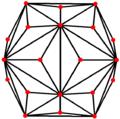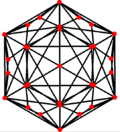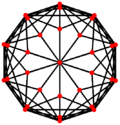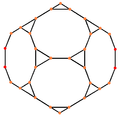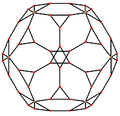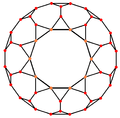## Kleetope

It can be seen as an icosahedron with triangular pyramids augmented to each face; that is, it is the Kleetope of the icosahedron. This interpretation is expressed in the name, triakis.## Other triakis icosahedra

This interpretation can also apply to other similar nonconvex polyhedra with pyramids of different heights:

•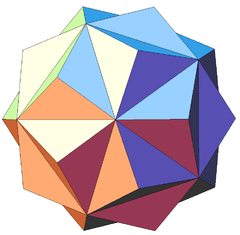First stellation of icosahedron, or small triambic icosahedron (sometimes called a triakis icosahedron)
•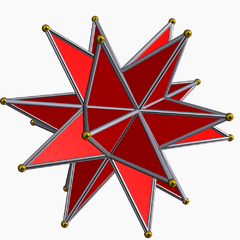Great stellated dodecahedron (with very tall pyramids)
•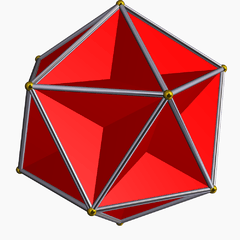Great dodecahedron (with inverted pyramids)

## Stellations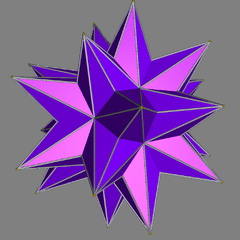The triakis icosahedron has numerous stellations, including this one.

## Related polyhedra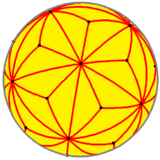Spherical triakis icosahedron

The triakis icosahedron is a part of a sequence of polyhedra and tilings, extending into the hyperbolic plane. These face-transitive figures have (*n32) reflectional symmetry.